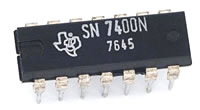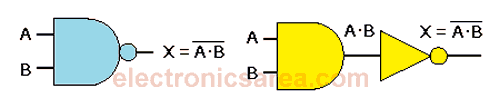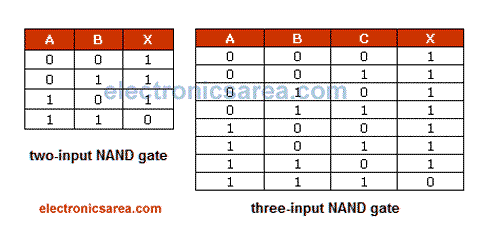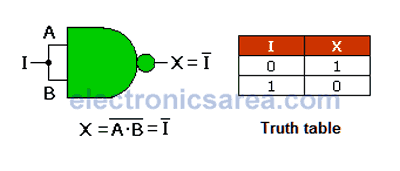# NAND Gate

Theoretically, a two inputs NAND gate can be implemented by cascading a two-input AND gate and a NOT gate (or inverter gate). See the image below.TTL 7400 NAND gate Integrated Circuit

As in the case of two-input AND gate, the same analysis can be done for the 3-input or more AND gates. As you can see, the X output is “0” only when all inputs are “1”.## Truth tables for the NAND gateNote: An interesting case of this type of gate. Like the NOR gate, on the first and last lines of the truth tables, the output X has the opposite value of the inputs.

In other words: A NAND logic gate can be used to obtain the behavior of a NOT gate

Although NAND gate seems to be the combination of two gates (one AND and one NOT gate), they are not. Actually, the NAND logic gate is not built by cascading an AND gate and a NOT gate, but it has an independent design.

We can create a NOT gate using a NAND gate. We do this by joining the inputs of the NAND gate, as seen on the next image.Analyzing the truth table of two-input NAND gate, we see that there are only two cases:

• When A = B = 0, I = 1
• When A = B = 1, I = 0
•
•
•
•
•
•
•
•
•
•
•
•
•
•
•
•
•
•